##CS660 Combinatorial Algorithms Fall Semester, 1996 AVL Trees

[To Lecture Notes Index]
San Diego State University -- This page last updated Oct 17, 1996## References

Data Structure Techniques, Standish, 1980, section 3.7.3

Handbook of Algorithms and Data Structures, Gonnet, 1984, section 3.4.1

`AVL Trees Slide # 1`

## Height-Balanced Trees

Height of a tree is the length of the longest path from the root to a leaf.

A binary search tree T is a height-balanced k-tree or HB[k]-tree, if each node in the tree has the HB[k] property

A node has the HB[k] property if the height of the left and right subtrees of the node differ in height by at most k.
Some HB Trees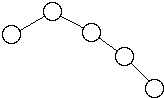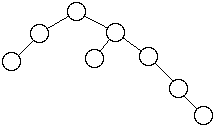`AVL Trees Slide # 2`

## AVL Trees

A HB tree is called an AVL tree.
Adel'son-Vel'skii and Landis first defined HB trees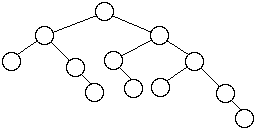Worst Case AVL Trees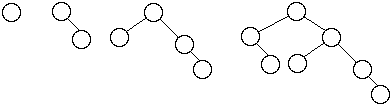Let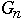= the number of nodes in worst case AVL tree of height n

We have: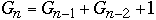`AVL Trees Slide # 3`
Fibonacci Numbers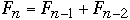,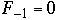,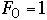n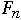0 1 1 1 2 1 2 4 2 3 7 3 4 12 5 5 20 8 6 33 13

We getBut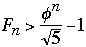where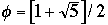So`AVL Trees Slide # 4`

Now take any AVL tree of height h with M nodes, we have

M >=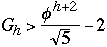So M + 2 >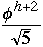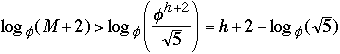h < 1..4404 lg(M + 2) - 0.328

Theorem ( Adel'son-Vel'skii and Landis 1962) The height of a balanced tree with N internal nodes always lies between lg (N +1 ) and 1..4404 lg(M + 2) - 0.328

`AVL Trees Slide # 5`

### Insertion into a AVL Tree

Some notationBalance InformationHeight of SubtreesInsert key as normal binary search tree
Restructure the tree if some node are unbalanced

`AVL Trees Slide # 6`
Case 0Inserting into Short Subtree
Balance Information ChangesNo Restructure of Tree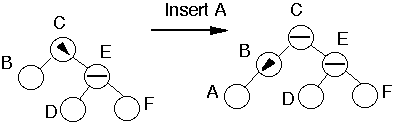General Case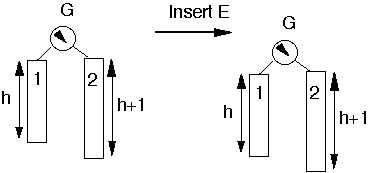or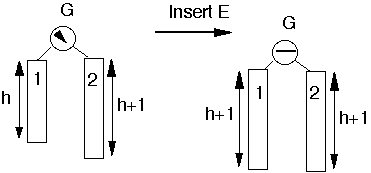`AVL Trees Slide # 7`
Case 1a
Insert into taller subtreeTaller subtree balancedTaller subtree on RightInsert into outer subsubtree
Example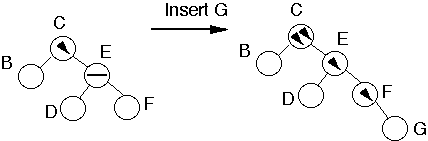Perform Single Rotation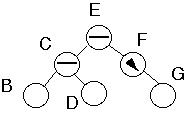Tree is balanced

`AVL Trees Slide # 8`
Need for Generalized Case 1a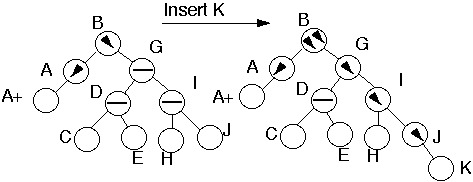`AVL Trees Slide # 9`
Case 1a
Insert into taller SubtreeTaller Subtree balancedTaller subtree on RightInsert into outer subsubtree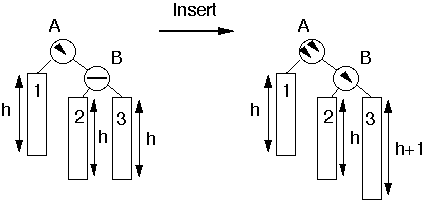Perform Single Rotation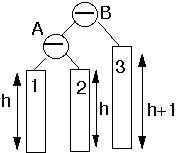Tree is balanced

`AVL Trees Slide # 10`
Case 1b
Insert into taller subtreeTaller subtree balancedTaller subtree on LeftInsert into outer subsubtree
This is symmetric to case 1a
Example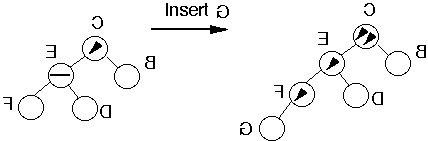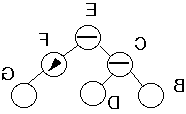`AVL Trees Slide # 11`
Case 2a
Insert into taller subtreeTaller subtree balancedTaller subtree on RightInsert into inner subsubtree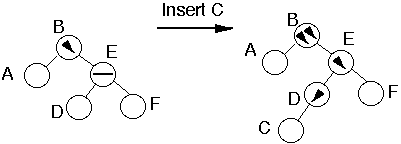Double Rotation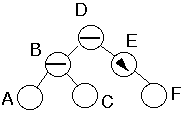`AVL Trees Slide # 12`
Case 2a
Insert into taller subtreeTaller subtree balancedTaller subtree on RightInsert into inner subsubtree
Insert into subtree 2 or 3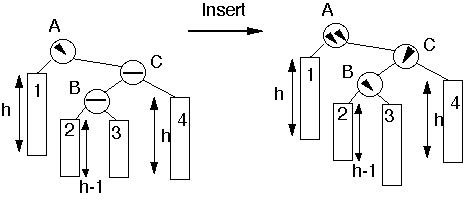Double Rotation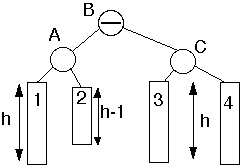`AVL Trees Slide # 13`
Case 2b
Insert into taller subtreeTaller subtree balancedTaller subtree on LeftInsert into inner subsubtree
This is symmetric to case 2a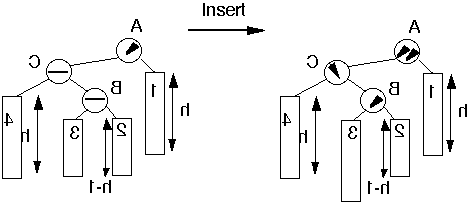`AVL Trees Slide # 14`
Case 3a
Insert into taller subtreeTaller subtree unbalancedTaller subtree on RightTaller subsubtree on RightInsert into outer subsubsubtree
Insert KReduces to Case 1aInsert KWe have covered all cases!

`AVL Trees Slide # 15`

### Deletions from an AVL Tree

Delete the node as in a binary search tree

The node deleted will be either a leaf or have just one subtree

Since this is an AVL tree if the deleted node has one subtree, then that subtree contains only one node

Traverse up the tree from the deleted node checking the balance of each node

`AVL Trees Slide # 16`
Case 1a
Traversing Up from Left subtreeDeletion reduced the height of the left subtreeNode has equal balance

Action:
Change balance of the node and stop
Have not effected the balance of any higher nodes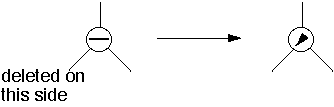Case 1b
Traversing Up from Right subtreeDeletion reduced the height of the Right subtreeNode has equal balance

Action:
Same as 1a

`AVL Trees Slide # 17`
Case 2a
Traversing Up from Left subtreeDeletion reduced the height of the left subtreeLeft subtree was greater then the right subtree

Action:
Change balance of the node and stop
May have effected the balance of any higher nodes, so continue up tree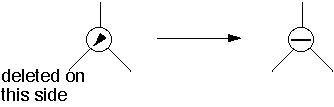Case 2b
Traversing Up from Right subtreeDeletion reduced the height of the Right subtreeRight subtree was greater then the Left subtree

Action:
Similar to 2a

`AVL Trees Slide # 18`
Case 3a
Traversing Up from Left subtreeDeletion reduced the height of the left subtreeRight subtree was greater then the Left subtree Left subtrees has equal subsubtrees

Action:
Perform single rotation, Adjust the balance
Have not effected the balance of any higher nodes
Stop hereSingle Rotation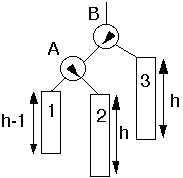`AVL Trees Slide # 19`
Case 4a
Traversing Up from Left subtreeDeletion reduced the height of the left subtreeRight subtree was greater then the Left subtree One or both of B subtrees has height h-1

Action:
Double rotation at B
May have effected the balance of any higher nodes, so continue up tree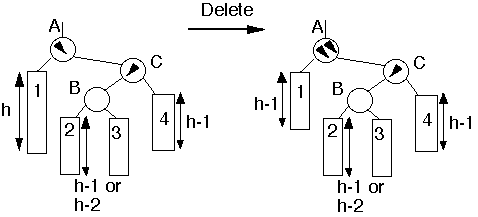Double Rotation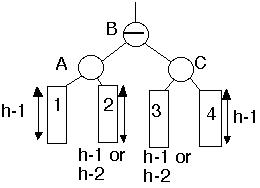`AVL Trees Slide # 20`
Case 5a
See picture

Action:
Single rotation at B
May have effected the balance of any higher nodes, so continue up tree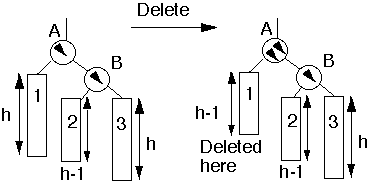`AVL Trees Slide # 21`

### Performance of AVL Trees

Theorem. An insertion into an AVL tree requires at most one rotation to rebalance a tree. A deletion may require lg(N) rotations to rebalance the tree.
Simulation Results AVL
 n C(n) E[ h(n) ] R(n) 5 2.2 3.0 0.213 10 2.907 4.0 0.318 50 4.930 6.947 0.427 100 5.889 7.999 0.444 500 8.192 10.923 0.461 1000 9.202 11.998 0.463 5000 11.555 14.936 0.465 10000 12.568 15.996 0.465
n = number of nodes in the tree
C(n) = average number of comparisons to find a key
E[ h(n) ] = expected height of tree
R(n) = average number of rotations per insertion/deletion